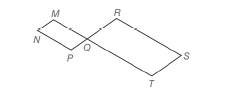Chapter 4.2, Problem 21E### Elementary Geometry for College St...

6th Edition
Daniel C. Alexander + 1 other
ISBN: 9781285195698

#### Solutions

Chapter
Section### Elementary Geometry for College St...

6th Edition
Daniel C. Alexander + 1 other
ISBN: 9781285195698
Textbook Problem
5 views

# In Exercises 19 to 24 , complete each proof. Given: M - Q - T and P - Q - R such that M N P Q and Q R S T are ▱ s Prove: ∠ N ≅ ∠ STo determine

To prove:

NS

Explanation

Given,

Given: M-Q-T and P-Q-R such that MNPQ and QRST are s.

Prove: NS

 PROOF Statements Reasons 1. MNPQ and QRST are ▱s. 1. Given 2. ∠MQP≅∠RQT 2. Vertical angles are equal 3. ∠MQP≅∠MNP∠RQT≅∠RST 3. Both pairs of opposite angles are congruent in a parallelogram 4. ∠MNP≅∠RSTOr ∠N≅∠S 4

### Still sussing out bartleby?

Check out a sample textbook solution.

See a sample solution

#### The Solution to Your Study Problems

Bartleby provides explanations to thousands of textbook problems written by our experts, many with advanced degrees!

Get Started